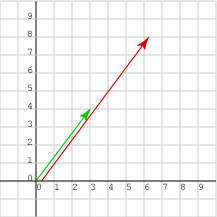Change the elements of this column matrix: (3, 4)T so that the vector it represents is twice as long and remains pointing in the same direction.

You could carefully work out the correct answer using what you already know. Or you could take a guess and see if it works:

```2 × (3, 4)T   =   (6, 8)T
```

# ScalingDoes the guess work?

• (new length)2 = 62 + 82   =   22 32 + 22 42   =   22 (32+42)   =   22 × (old length)2
• So, taking roots, (new length) = 2 × (old length)
• By similar triangles, the direction is the same.

Or you could notice: new direction   =   arc tan( 8/6 )   =   arc tan( 4/3 )   =   old direction.

It looks like the guess worked.

When talking about vectors, a real number is sometimes called a scalar.

Scaling a geometrical vector means keeping its orientation the same but changing its length by a scale factor. It is like changing the scale of a picture; the objects expand or shrink, but the directions remain the same.

If a vector is represented by a column matrix   (x, y)T   then scaling by the a number multiplies each element:

```a(x, y)T  =  (ax, ay)T
```

This works for 3 dimensional vectors also: If v is represented by (x, y, z)T then av is represented by (ax, ay, az)T.

### QUESTION 2:

What is 0.5×(36.4, -18.9)T ?